# CZ flag

What percentage of the Czech flag is made up of blue, white and red textiles?

p1 =  37.5 %
p2 =  37.5 %
p3 =  25 %

### Step-by-step explanation:

${p}_{2}={p}_{1}=37.5=37.5\mathrm{%}$
${p}_{3}=100-{p}_{1}-{p}_{2}=100-37.5-37.5=25\mathrm{%}$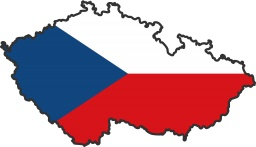Did you find an error or inaccuracy? Feel free to write us. Thank you!Tips to related online calculators

#### You need to know the following knowledge to solve this word math problem:

We encourage you to watch this tutorial video on this math problem:

## Related math problems and questions:

• Cllub flag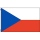The children had the club flag sewn on. The red color covered 2/20 of the total area of the flag, the blue area a fifth, the yellow 19% of the total area. What% is the green area occupied?
• ExpansionIf one side of the rectangle is larger 4-times and second 2-times, what percentage increases the area of rectangle?
• Here are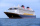Here are white, blue, and red boats in a marina. Two-thirds of the boats in the marina are white, 4/7 of the remaining boats are blue, and the rest are red. If there are 15 red boats, how many boats are in the marina? Which tape diagram represents how man
• CandiesThere are red, blue and green candies in bad. Red to green is in 6:11 ratio and blue to red in a 7: 5 ratio. In what proportion are blue to green candies?
• Percentage and rectangleAbout what percentage increases perimeter and area of a rectangle if both the sides 12 cm and 10 cm long we increase by 20%?
• Components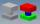In the box are 8 white, 4 blue and 2 red components. What is the probability that we pull one white, one blue and one red component without returning?
• The golfThe golf ball with a diameter of 43 mm is packed in a cube-shaped box. What percentage of the box volume is made up of a ball?
• What percentageWhat percentage is 12 km out of 80 km?
• The flag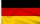The flag should consist of 3 different colored stripes. Available colors: white, red, blue, green and yellow. Specify: A) number of all flags B) number of flags with a blue stripe C) number of flags with a blue stripe in the middle D) the number of flags
• Hop-gardenThe length of the rectangular hop garden Mr. Smith increased by 25% and its width by 30%. What is the percentage change in area of hop garden?
• PC disksPeter has 45 disks in three colors. One-fifth of the disks are blue, red are twice more than the white. How much is blue, red and white disks?
• Percentage of waste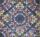In a square plate with side 75 cm we cut 4 same circles. Calculate the percentage of waste.
• Climb in percentageThe height difference between points A and B is 475 m. Calculate the percentage of route climbing if the horizontal distance places A, B is 7.4 km.
• The blueThe blue and red coats originally cost the same. Then red prices rose by 27% and blue prices fell by 87%. Then their price differed by CZK 300. How much did the originally blue coat cost?
• Company logoThe company logo consists of a blue circle with a radius of 4 cm, which is an inscribed white square. What is the area of the blue part of the logo?
• Irregular pentagon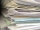A rectangle-shaped, 16 x 4 cm strip of paper is folded lengthwise so that the lower right corner is applied to the upper left corner. What area does the pentagon have?
• The length 2The length of a rectangle is 12y2 – 15y + 8 and the width is 7y – 11. Find the area of the rectangle